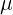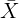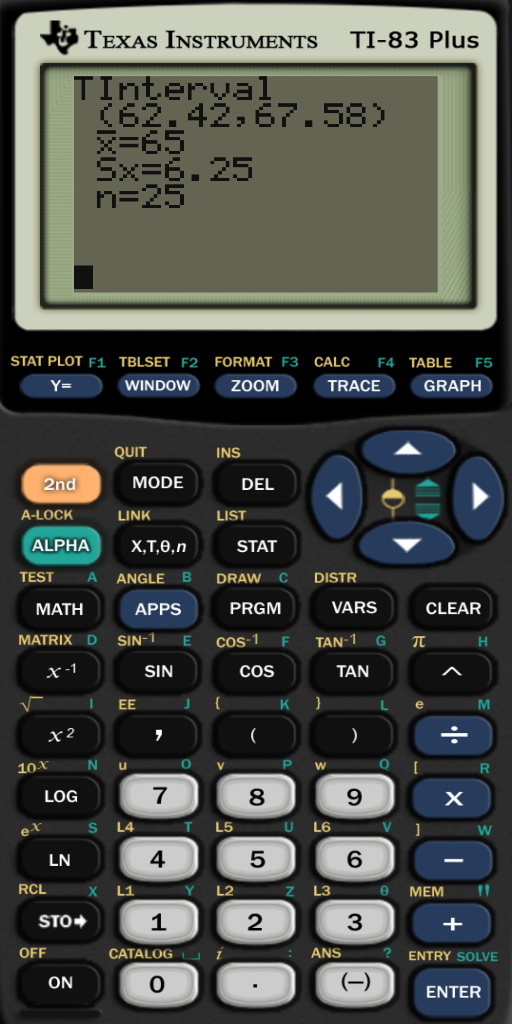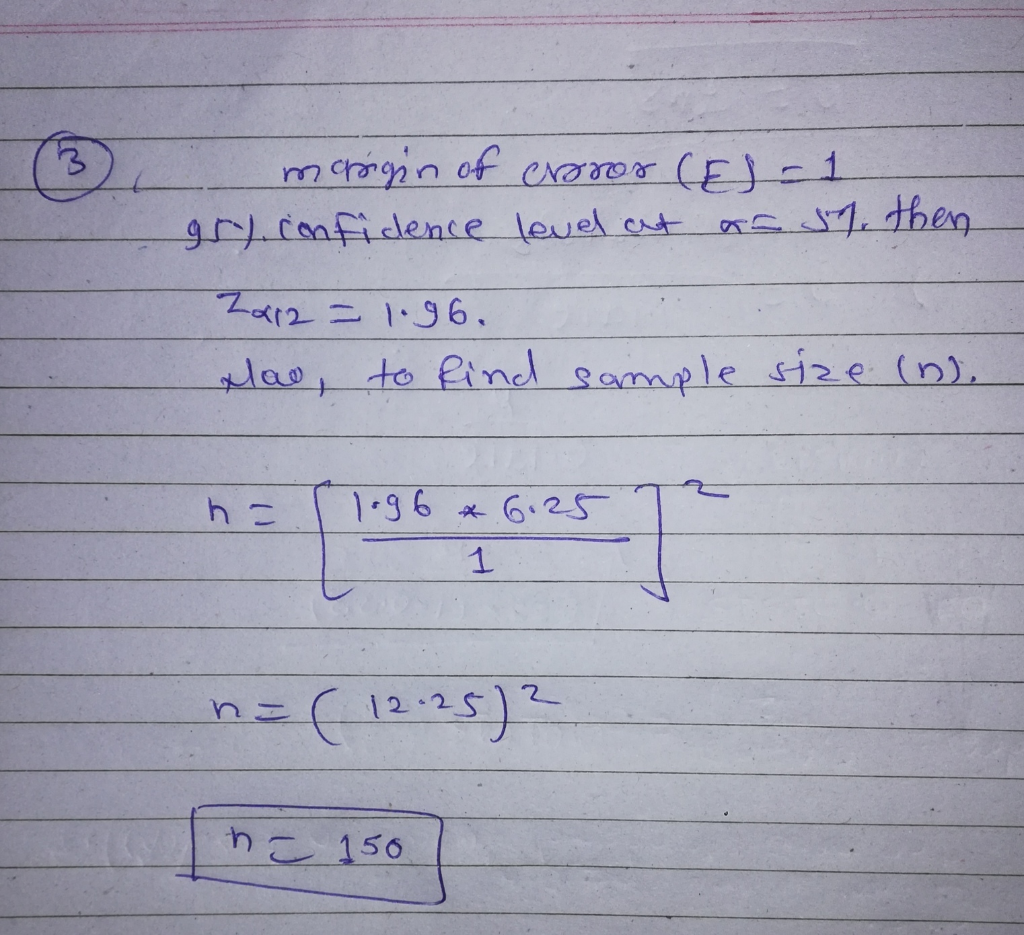##### Question

In: Statistics and Probability

# A survey of 25 randomly selected psychiatrists found an average hourly wage (including benefits) of $65.00... A survey of 25 randomly selected psychiatrists found an average hourly wage (including benefits) of$65.00 per hour. The sample standard deviation was $6.25 per hour. 1. What is the population mean? What is the best estimate of the population mean? 2. Develop a 99% confidence interval for the population mean wage (including benefits) for these psychiatrists. 3. How large a sample is needed to assess the population mean with an allowable error of$1.00 at 95% confidence?

## Solutions

##### Expert Solution

1)

Population mean () = sample mean () =65.00

The best estimate of a population mean is the sample mean.

The most fundamental point and interval estimation process involves the estimation of the population mean.

2)

Here using ti-83 calculator.## Related Solutions

##### 2) A survey of 25 randomly selected customers found that their average age was 31.84 years...
2) A survey of 25 randomly selected customers found that their average age was 31.84 years with a standard deviation of 9.84 years. a. What is the standard error of the mean? b. How would the standard error have changed if the sample size had been 100 instead of 25 (assuming that the mean and standard deviations were the same)? c. How many degrees of freedom would the t-statistic have for this set of data? d. What would the critical...
##### A survey of 25 randomly selected customers found the ages shown (in years). The mean is...
A survey of 25 randomly selected customers found the ages shown (in years). The mean is 32.64 years and the standard deviation is 9.97 years. ​a) What is the standard error of the​ mean? ​b) How would the standard error change if the sample size had been 400 instead of 25​? ​(Assume that the sample standard deviation​ didn't change.)
##### A survey of 25 randomly selected customers found the ages shown​ (in years). The mean is...
A survey of 25 randomly selected customers found the ages shown​ (in years). The mean is 32.24 years and the standard deviation is 9.55 years.​ 30 41 48 36 20 36 37 36 26 27 17 23 39 35 21 10 31 42 32 41 43 28 47 25 35 a) Construct a 90% confidence interval for the mean age of all​ customers, assuming that the assumptions and conditions for the confidence interval have been met. ​b) How large is...
##### A survey of 25 randomly selected customers found the ages shown​ (in years). The mean is...
A survey of 25 randomly selected customers found the ages shown​ (in years). The mean is 31.52 years and the standard deviation is 10.24 years. ​a) Construct a 95​% confidence interval for the mean age of all​ customers, assuming that the assumptions and conditions for the confidence interval have been met. ​b) How large is the margin of​ error? ​c) How would the confidence interval change if you had assumed that the standard deviation was known to be 11.0 ​years?...
##### A survey of 25 randomly selected customers found the ages shown​ (in years). The mean is...
A survey of 25 randomly selected customers found the ages shown​ (in years). The mean is 33.16 years and the standard deviation is 9.33 years.​ a) Construct a 95​% confidence interval for the mean age of all​ customers, assuming that the assumptions and conditions for the confidence interval have been met. ​b) How large is the margin of​ error? ​c) How would the confidence interval change if you had assumed that the standard deviation was known to be 10.0 ​years?...
##### A survey of 25 randomly selected customers found the ages shown​ (in years). The mean is...
A survey of 25 randomly selected customers found the ages shown​ (in years). The mean is 32.04 years and the standard deviation is 9.87 years. ​a) Construct a 80% confidence interval for the mean age of all​ customers, assuming that the assumptions and conditions for the confidence interval have been met. ​b) How large is the margin of​ error? ​c) How would the confidence interval change if you had assumed that the standard deviation was known to be 10.0 ​years?
##### A survey of 25 randomly selected customers found the ages shown​ (in years). The mean is...
A survey of 25 randomly selected customers found the ages shown​ (in years). The mean is 31.96 years and the standard deviation is 10.12 years. ​a) Construct a 95​% confidence interval for the mean age of all​ customers, assuming that the assumptions and conditions for the confidence interval have been met. ​b) How large is the margin of​ error? ​c) How would the confidence interval change if you had assumed that the standard deviation was known to be 11.0 ​years?
##### A survey of 25 randomly selected customers found the ages shown (in years). The mean is...
A survey of 25 randomly selected customers found the ages shown (in years). The mean is 32.44 years and the standard deviation is 10.17 years. a) Construct a 90% confidence interval for the mean age of all customers, assuming that the assumptions and conditions for the confidence interval have been met. b) How large is the margin of error? c) How would the confidence interval change if you had assumed that the standard deviation was known to be 11.0 years?
##### A survey of 25 randomly selected customers found the ages shown​ (in years). The mean is...
A survey of 25 randomly selected customers found the ages shown​ (in years). The mean is 32.32 years and the standard deviation is 10.66 years.​ a) Construct a 99% confidence interval for the mean age of all​ customers, assuming that the assumptions and conditions for the confidence interval have been met. ​b) How large is the margin of​ error? ​c) How would the confidence interval change if you had assumed that the standard deviation was known to be 11.0 ​years?
##### Wage and unions. Listed below are sample characteristics from a 1987 survey that examines average hourly...
Wage and unions. Listed below are sample characteristics from a 1987 survey that examines average hourly wage rates for union and non-union workers. Nonunion: ?̅?? = 11.47; ???= 1206; ???= 6.58 Union: ?̅? = 12.19; ??= 376; ?? = 4.77 a. What is the difference in average hourly wages between union and nonunion workers? b. Construct a 95% confidence interval around this difference. c. Test the null hypothesis that there is no difference in wages across the two groups.